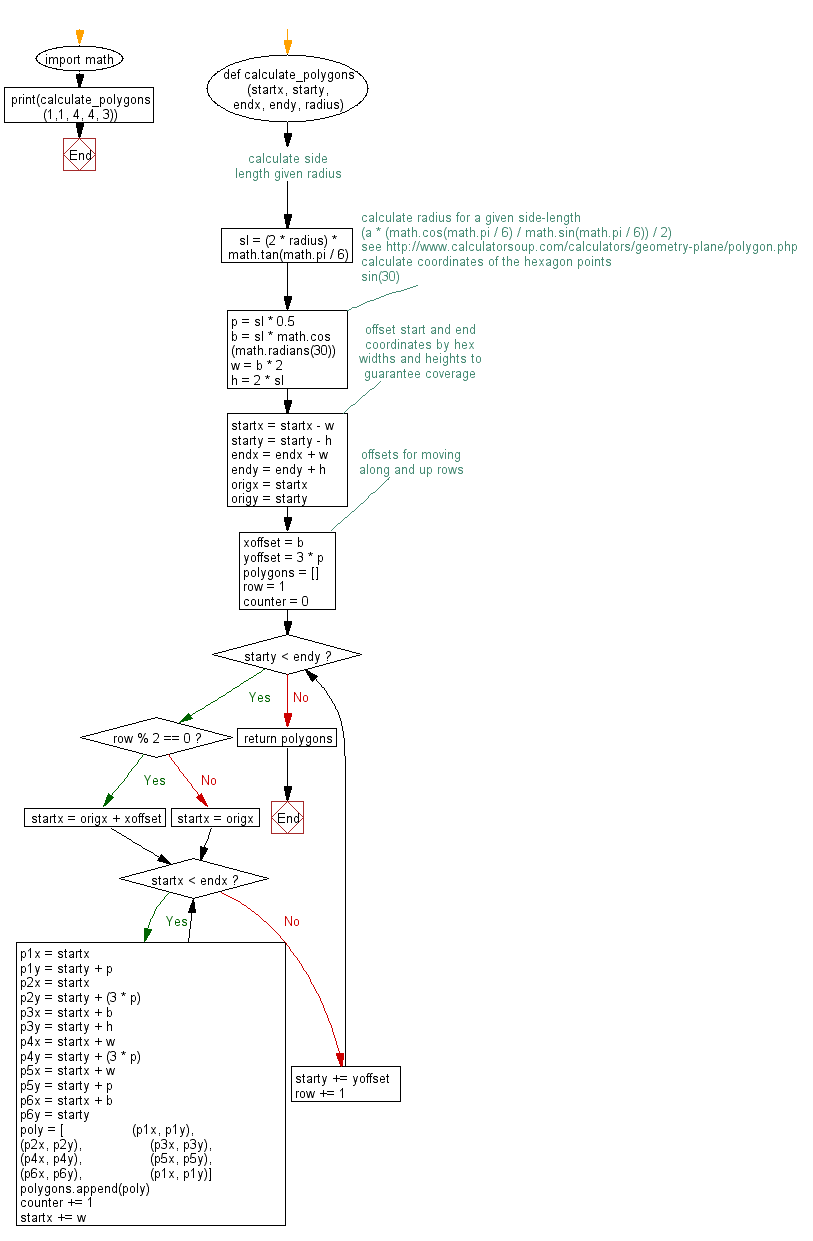﻿ Python Math: Calculate a grid of hexagon coordinates of the given radius given lower-left and upper-right coordinates - w3resource# Python Math: Calculate a grid of hexagon coordinates of the given radius given lower-left and upper-right coordinates

## Python Math: Exercise-62 with Solution

Write a Python program to calculate a grid of hexagon coordinates of the given radius given lower-left and upper-right coordinates. The function will return a list of lists containing 6 tuples of x, y point coordinates. These can be used to construct valid regular hexagonal polygons.

Sample Solution:-

Python Code:

``````#https://gist.github.com/urschrei/17cf0be92ca90a244a91
import math
def calculate_polygons(startx, starty, endx, endy, radius):
# calculate side length given radius
sl = (2 * radius) * math.tan(math.pi / 6)
# calculate radius for a given side-length
# (a * (math.cos(math.pi / 6) / math.sin(math.pi / 6)) / 2)
# see http://www.calculatorsoup.com/calculators/geometry-plane/polygon.php

# calculate coordinates of the hexagon points
# sin(30)
p = sl * 0.5
w = b * 2
h = 2 * sl

# offset start and end coordinates by hex widths and heights to guarantee coverage
startx = startx - w
starty = starty - h
endx = endx + w
endy = endy + h

origx = startx
origy = starty

# offsets for moving along and up rows
xoffset = b
yoffset = 3 * p

polygons = []
row = 1
counter = 0

while starty < endy:
if row % 2 == 0:
startx = origx + xoffset
else:
startx = origx
while startx < endx:
p1x = startx
p1y = starty + p
p2x = startx
p2y = starty + (3 * p)
p3x = startx + b
p3y = starty + h
p4x = startx + w
p4y = starty + (3 * p)
p5x = startx + w
p5y = starty + p
p6x = startx + b
p6y = starty
poly = [
(p1x, p1y),
(p2x, p2y),
(p3x, p3y),
(p4x, p4y),
(p5x, p5y),
(p6x, p6y),
(p1x, p1y)]
polygons.append(poly)
counter += 1
startx += w
starty += yoffset
row += 1
return polygons

print(calculate_polygons(1,1, 4, 4, 3))
```
```

Sample Output:

```[[(-5.0, -4.196152422706632), (-5.0, -0.7320508075688767), (-2.0, 1.0), (1.0, -0.7320508075688767), (1.0, -4.1
96152422706632), (-2.0, -5.928203230275509), (-5.0, -4.196152422706632)], [(1.0, -4.196152422706632), (1.0, -0
.7320508075688767), (4.0, 1.0), (7.0, -0.7320508075688767), (7.0, -4.196152422706632), (4.0, -5.92820323027550
9), (1.0, -4.196152422706632)], [(7.0, -4.196152422706632), (7.0, -0.7320508075688767), (10.0, 1.0), (13.0, -0
.7320508075688767), (13.0, -4.196152422706632), (10.0, -5.928203230275509), (7.0, -4.196152422706632)], [(-2.0
------
), (10.0, 14.85640646055102), (10.0, 11.392304845413264), (7.0, 9.660254037844387), (4.0, 11.392304845413264)]
]
```

Flowchart:## Visualize Python code execution:

The following tool visualize what the computer is doing step-by-step as it executes the said program:

Python Code Editor:

Have another way to solve this solution? Contribute your code (and comments) through Disqus.

What is the difficulty level of this exercise?

Test your Programming skills with w3resource's quiz.

﻿

```>>> students = [{'name': 'John', 'score': 98}, {'name': 'Mike', 'score': 94}, {'name': 'Jennifer', 'score': 99}]